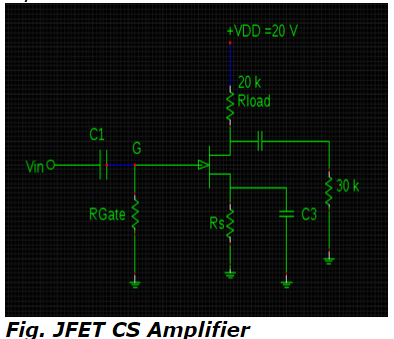# Common Source JFET amplifier Gain - MCQs with answers

## Common Source JFET amplifier Gain - MCQs with answers

Q1. Which phenomenon occurs between the input signal at the gate and output signal at the drain in the operational mode of common source JFET amplifier?

a. Phase Inversion
b. Frequency Inversion
c. Amplitude Inversion
d. Pulse-angle Inversion

Q2. The tremendous increase in the value of input capacitance usually considered as noxious for high-frequency operations of common source JFET amplifier, is an ultimate outcome of _______.

a. Aliasing Effect
b. Carson's Effect
c. Miller's Effect
d. Barkheussan's Effect

Q3. What is the voltage gain of below drawn JFET CS amplifier circuit whose transconductance and drain to source resistance values are 2000 μS & 200 k Ω respectively?a. -56.56 V
b. 56.56 V
c. -36.36 V
d. 36.36 V